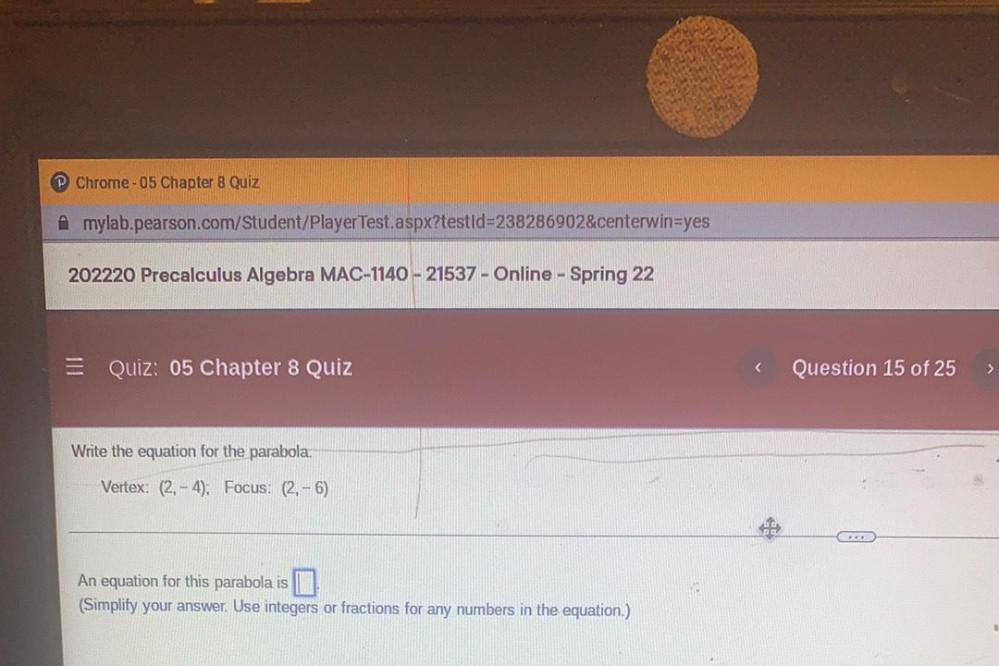Question:

# Chrome-05 Chapter 8 Quiz Amylab.pearson.com/Student/Player Test.aspx?testId=238286902&centerwin=yes 202220 Precalculus Algebra MChrome-05 Chapter 8 Quiz Amylab.pearson.com/Student/Player Test.aspx?testId=238286902&centerwin=yes 202220 Precalculus Algebra MAC-1140 - 21537 - Online - Spring 22 = Quiz: 05 Chapter 8 Quiz Question 15 of 25 > Write the equation for the parabola Vertex: (2. - 4). Focus: (2.-6) An equation for this parabola is (Simplify your answer. Use integers or fractions for any numbers in the equation.)